# Using Video Measure Analysis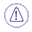#### Licensing

Stock Analysis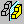Video Measure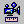is only available if you have an NC Manufacturing Verification product license.This procedure describes how to do measure analysis. With this functionality, you can make the following types of measurements:
• distance and angle between planar surfaces and edges
• distance and angle between centerlines of circular entities
• distance and angle between circular entities and planes or edges
• distances between points and other supported entities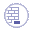Click Stock Analysisin either the Process Simulation toolbar (while the simulation is paused) or on the Stock Management toolbar, when no simulation is in process.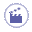1. In the Analysis dialog box, click Video Measure.

 The Video Measure dialog box appears.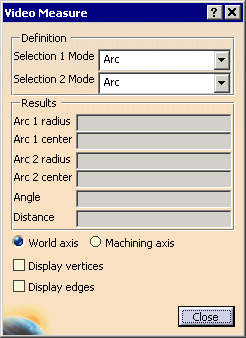2. Select the Display vertices and Display Edges check boxes to display all the edges and vertices of the solid.

3. Select the desired option in both the Selection 1 Mode and Selection 2 Mode list.

The options are:
• Arc
• Plane
• Edge
• Point
These options can selected in any combination. Below are some of the possible selection combinations:
 Arc/Arc Measures the distance between two arc center axes. Plane/Plane Measures the distance and angle between two planes Arc/Plane or Plane/Arc Measures the distance between an arc center axis and a plane or vice versa. Edge/Edge Measures the distance and angle between two parallel edges. If they are not parallel, only the angle between them is measured. Point/Point Measures the distance between two points. The coordinates of both points are also displayed. Edge/Arc Measures the distance and angle between an edge and an arc center axis if the edge is parallel to the arc center axis. If they are not parallel, only the angle between them is measured. Edge/Plane Measures the distance and angle between an edge and a plane if the edge is parallel to the plane. If they are not parallel, only the angle between them is measured. Edge/Point Measures the shortest distance between an edge and a vertex. The coordinates of the point on the edge from which the minimum distance is calculated is also displayed. Point/Arc Measures the shortest distance between the arc center axis and the vertex. Point/Plane Measures the shortest distance between a point and a plane